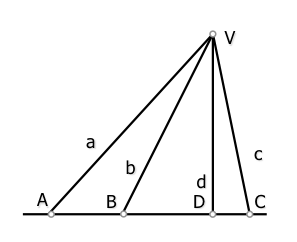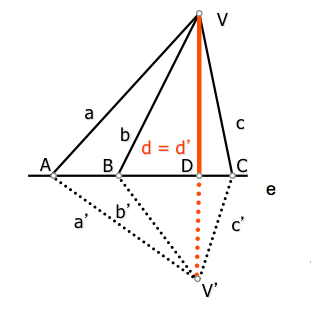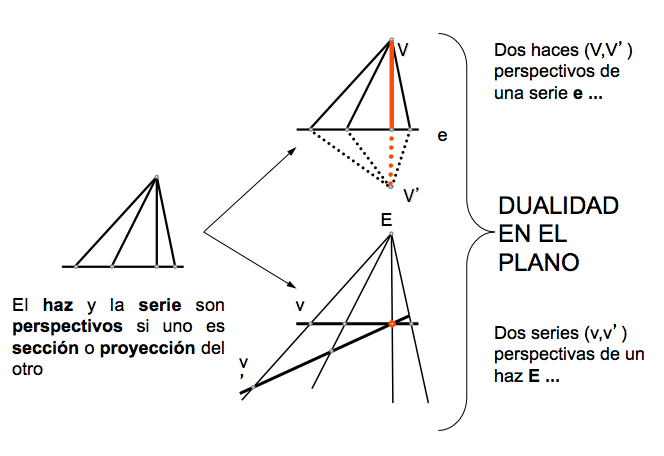# Geometría proyectiva: PerspectivityProjective foundations are based on the definitions of "ordered triples of elements"And “quaternions that establish the concept of cross ratio“, and relationships called “perspectives” between elements of identical or different nature.

These perspectives relations, that will be used in determining projections representation systems, defined from two projective operators:

• Projection
• SectionProject from a vertex V.. a rectilinear series s, formed by a set of aligned points A, B, C …. obtain the beam is straight to, b, c … vertex at center of projection V...

Sections a straight s a bundle of concurrent lines to, b, cVertex V.., is to obtain the rectilinear series of aligned points A, B, C ….straight based s.

If we take these four elements of geometric shapes (series and make straight lines) we can determine quadruples of elements that have a specific value of your property, as we have defined the study quadruples of items ordered. This value, as discussed, is the same in the case of quaternions in dotted lines and if a projection or sectional are of the other. Namely:

(abcd) = (ABCD)

The cross ratio of four lines of a single beam, is the number of points obtained as a straight section that does not contain any vertex of the beam.

Similarly we have the dual theorem:

The cross ratio of four points of the same series, It is obtained as the straight projection from any point that does not contain the base of the series.

We say that the line and the beam are perspectival section or if you are projecting another.

We therefore first definition of perspectivity between forms of the same class, but of a different nature (Vs straight points).

Can we establish a concept perspective similar between two beams or two series?

## Beam straight perspectivity.

We can give different definitions of perspectivity between two coplanar beams straight.

Two beams of different vertices straight, V.. and V..‘, perspective are each other, as may be obtained as a projection of a common set.and: perspectival axis

When projecting from the vertices V and V’ points (ABC…) perspectival a series two beams having a common beam are obtained ( d = d’), so that it holds that quaternions related elements are identical:

(abcd) = (ABCD) = (a’b’c’d’)

• Beams of vertices V and V 'are perspectival with perspectival axis straight and support (base) Series projecting.
• Each beam line vertex V and its beam homologous vertex V ' cut on that axis.
• The element d = d 'containing the base V and V' beams, is a dual element

## Perspectivity between sets of points.

As in every theorem we can establish in projective geometry, can get one dual changing the element that determines. So, in the case of a series of points spectivity find a definition similar to that given for the straight beams:

Of the series of points of different bases, s and s‘, prospects are each other, as may be obtained as a single beam section.V..: center perspective

On sectioning by straight r and r’ rays (abc…) Do two perspectives of a series that have a common point are obtained ( D = D’), so that it holds that quaternions related elements are identical:

(ABCD) = (abcd) = (A’B’C’D’)

• The basis sets r and r'Prospects are with center perspective point V support (vertex) beam that sectioned.
• Each point in the series of basis ry their homologous series base r’ projected that center.
• The element D = D 'containing the base r r' series, is a dual element

## Duality in the plane

We see therefore that there is a duality between the properties and theorems that link the point and straight from the plane by, may be obtained from each other by changing the words point and plane statements, and projection operations by section.

In summary of the above, we can present a simple diagram that will simplify the above. We will see later the importance of relationships to understand perspectives projective.